#### A grid-based approach

Perhaps the most straightforward way to numerically compute probabilistic I-states is to approximate probability density functions over a grid and use numerical integration to evaluate the integrals in (11.57) and (11.58).

A grid can be used to compute a discrete probability distribution that approximates the continuous probability density function. Consider, for example, using the Sukharev grid shown in Figure 5.5a, or a similar grid adapted to the state space. Consider approximating some probability density function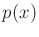using a finite set,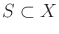. The Voronoi region surrounding each point can be considered as a bucket'' that holds probability mass. A probability is associated with each sample and is defined as the integral ofover the Voronoi region associated with the point. In this way, the samples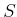and their discrete probability distribution,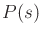for all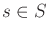approximateover. Let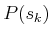denote the probability distribution over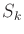, the set of grid samples at stage.

In the initial step,is computed fromby numerically evaluating the integrals of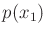over the Voronoi region of each sample. This can alternatively be estimated by drawing random samples from the densityand then recording the number of samples that fall into each bucket (Voronoi region). Normalizing the counts for the buckets yields a probability distribution,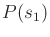. Buckets that have little or no points can be eliminated from future computations, depending on the desired accuracy. Let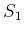denote the samples for which nonzero probabilities are associated.

Now suppose that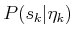has been computed overand the task is to compute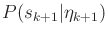given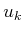and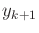. A discrete approximation,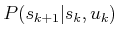, to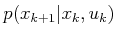can be computed using a grid and buckets in the manner described above. At this point the densities needed for (11.57) have been approximated by discrete distributions. In this case, (11.38) can be applied overto obtain a grid-based distribution over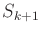(again, any buckets that do not contain enough probability mass can be discarded). The resulting distribution is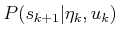, and the next step is to consider. Once again, a discrete distribution can be computed; in this case,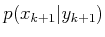is approximated by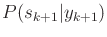by using the grid samples. This enables (11.58) to be replaced by the discrete counterpart (11.39), which is applied to the samples. The resulting distribution,, represents the approximate probabilistic I-state.

Steven M LaValle 2020-08-14